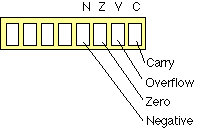# The Integer Unit

The Integer Unit (sometimes called the "Arithmetic and Logic Unit" (ALU)) is the central part of the processor: it is basically a combinational logic element, that can do both arithmetic and logical operations on integer numbers: for example, two words can be added, subtracted, ANDed or ORed together. The operation to be executed is controlled by the values on the control inputs to the Integer Unit supplied by the Dispatch Unit.

The Integer Unit deals with either bytes or words (a word is usually 2 or 4 bytes): in general it takes two eight bit numbers, and gives one eight bit answer, or two words and gives another word as an answer. One of the two words or bytes may be a location in memory, the other is usually the contents of one of the registers, or else is eight bits actually stored in the instruction. The latter is called a "literal". (In assembler we normally write a literal value by preceding it with a hash "#"). The output of the Integer Unit is either written back to memory, or else it is written back to the the register where the data came from.The control bits (or commands), which come from the Dispatch Unit, determine what happens. The dispatch unit processes the instrcution held in the instruction register, and decodes this to generate the control bits. These bit dictate whether the input is taken from a register or from memory; what logical or arithmetic operation is performed; whether the data is a byte or a word; and where the output is written. The instruction also contains the address of the register involved, or the "literal" value to be used.

####The Integer Unit also has some "status" outputs: for example, if the answer is zero, the Z bit is set to 1; and if there was a "carry" the carry bit is set to 1. The new values of the status bits are stored in the Integer Unit status register after completion of each instruction. Many instructions modify only some status bits, leaving the value of the other bits unchanged.

#### Executing Instructions in the Integer Unit

Here are some of the elementary instructions which can be done (I am spelling them out in a longer form than they are usually written, the mnemonic in brackets indicates the more usual short-hand form).

• Add contents of memory location W to contents of register r0, and write answer in register r0
• C: register int r; int W; r= r+w;
• Add contents of register r0 to contents of register r1, and write answer in register r0
• C: register int r0,r1; r0=r0+r1;
• Take contents of register r0, write to location W in memory
(The output of the ALU is simply equal to one of its inputs.)
• C: int w; register int 0; W=r0;
• Assembler: MOV W,r0
• Take contents of register r2, write to r3
(The output of the ALU is simply equal to one of its inputs.)
• C: register int r2,r3; r3=r2;
• Assembler: MOV r3, r2
• Increase contents of register r0 by 1, write answer in register r0
• C: register int r0; r0++;
• Assembler: INC r0
• Decrease contents of register r4 by 1, write answer in register r4
• C: register int r4; r4--;
• Assembler: DEC r4
• Shift right the value of register r0 by one bit, write answer back in r0
• C: register int r0; r0=r0>>1;
• Assembler: SHFTL r0,1
• Logical AND contents of register r4 and register r1 (bit by bit); write in r4
• C: register int r4,r1; r4=r1&r4;
• Assembler: AND r4, r1
• Add literal eight bit value, V, to register r6; write answer in r6
• C: register char r6; const V; r6=r6+V;
• Write literal eight bit value, V, to register r6
• C: register char r6; const V; r6=V;
• Assembler: MOV.B r6, #V

Note that a small number of choices define all these possibilities. Each instruction has three parts: an operation code (opcode) which specifies what the Integer Unit is to do; a size which specifies how many bits are to be used (in many cases the default (usual) size is a whole word); a destination which is where the result of the operation is returned (the destination is usually also read into the Integer Unit for most opcodes); and a source address, which specifies where the data is to come from.

A list of common instructions follows:

Unary operators (operate on one register)
= assign value (move)
~a bit-wise inversion (1's complement)
-a negation (2's complement)
a++ increment a (add 1 to a)
a-- decrement a (subtract 1 from a)
Logical operators
a & b Bit-wise logical and of a and b
a ^ b Bit-wise exclusive OR (XOR)
a | b Bit-wise OR
a >> n Shift a right n bits (a divideed by 2 to the power n)
a << n Shift a left n bits (a multiplied by 2 to the power n)
Arithmetic Operators
a +b add a to b
a - b subtract b from a
a * b a multiplied by b
a / b a divided by b